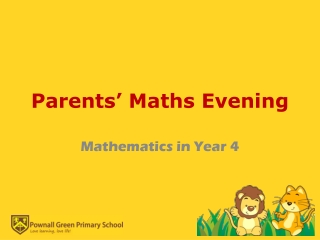DownloadDownload PresentationParents’ Maths Evening

# Parents’ Maths Evening

Télécharger la présentation## Parents’ Maths Evening

- - - - - - - - - - - - - - - - - - - - - - - - - - - E N D - - - - - - - - - - - - - - - - - - - - - - - - - - -
##### Presentation Transcript

1. Parents’ Maths Evening Mathematics in Year 4

2. Aims • Develop understanding of maths in Year 4 • Provide strategies to support your child at home

3. Focus • Fluency in numbers • Problem solving • Mathematical reasoning

4. Exploding Numbers How many ways can you make 48?

5. Fluency HELPING YOUR CHILD WITH MULTIPLICATION TABLES

6. 2x tables – tips Products are EVEN DOUBLE HALVE

7. 3x table – tips If the digits add up to 3, 6, or 9, then it's in the 3 times table

8. 4x table – tips All the products are EVEN DOUBLE and DOUBLEAGAIN If the last 2 digits of a number are a multiple of 4, then the number is Reverse the calculation if that makes it easier .

9. 5x and 10x tables – tips Multiples of 5 end in a 5 or a 0 Multiples of 10 always end in a 0

10. 6x table – tips • All the products are EVEN and a multiple of 3 • X3, thenDOUBLE it • Reverse the calculation if that makes it easier

11. 7x table - tips 7 x 8 = 56. Just remember - 5,6,7,8Reverse the calculation 4 rows of 7 (4 x 7). Count them up - there are 28. o o o o o o o    o o o o o o o    o o o o o o o    o o o o o o o It is the same as 7 x 4 - 7 rows of 4.o o o o    o o o o    o o o o    o o o o    o o o o    o o o o    o o o o

12. 8x table – tips The products are always EVEN X4 then DOUBLE it The unit digits have a regular pattern - they go down in 2s

13. 9x table – tips The tens go up but the units go down 1 x 9 = 9     2 x 9 = 18     3 x 9 = 27     4 x 9 = 36     5 x 9 = 45     6 x 9 = 54 The digits of the products add up to 918 = 1 + 8 = 9    27 = 2 + 7 = 9    36 = 3 + 6 = 9

14. The Finger Trick 7 X 9 Hold out all 10 fingers, and lower the 7th finger. There are 6 fingers to the left and 3 fingers on the right. The answer is 63.

15. When to practise multiplication tables WHENEVER WHEREVER LITTLE AND OFTEN REAL-LIFE CONTEXTS REVISIT

16. Fluency in Numbers • Multiplication tables: Fast 50 • Strategies for multiplication tables: • in car/ shower, • sergeant 7 • Rhymes • music

17. Fluency in Numbers • Mental strategies: • partitioning • doubling and halving • near doubles • round to nearest multiple of ten/ hundred/ thousand and adjust

18. Telling the Time • Analogue • Quarter past, half past, quarter to, o’clock • 5 past, 10 past, 5 to. 10 to • Symmetrical • Apps: Mr Wolf Telling the Time • http://resources.woodlands-junior.kent.sch.uk/maths/measures.htm

19. Telling the Time • Problem solving out and about

20. Formal written methods • Column additionmm • Column subtraction • Short column multiplication – moving on from repeated addition • Short bus stop division – moving on from repeated subtraction

21. Problem Solving RUDECAT • Read and understand the problem • Underline Key information • Decide what you need to work out ( which operation?) • Estimate • Calculate • Answer in a sentence • Take time to check your answer

22. Mathematical Reasoning When first encountering a new challenge to make use of relevant prior knowledge • 'type' of problem • particular calculation method that could prove useful.

23. When there are a range of possible starting points • Trial and error • Prior knowledge – patterns and relationships between numbers

24. When there is missing information • Recognise that information is missing • Figure out what it is that we need to know • Draw on our existing knowledge

25. Mathematical reasoning • Number triangles – p5

26. I have fifteen cards numbered 1− 15  I put down seven of them on the table in a row. The numbers on the first two cards add to 15  The numbers on the second and third cards add to 20  The numbers on the third and fourth cards add to 23  The numbers on the fourth and fifth cards add to 16  The numbers on the fifth and sixth cards add to 18  The numbers on the sixth and seventh cards add to 21 What are my cards? Can you find any other solutions? How do you know you've found all the different solutions?

27. Websites to help support your child • MyMaths website provides a range of online lessons, resources and games • Woodlands Junior School has many activities and games for different maths concepts • BBC Schools website provides useful tips, ideas and resources • Mad4Mathsis a maths interactive site with advice for parents as well as games • A Maths Dictionary for Kids – vocabulary • nrich is a website with lots of problem solving tasks and helps children to develop mathematical reasoning

28. Exit Ticket • What have you found useful about tonight’s workshop • Blu-tack it to the whiteboard • Show how confident you feel helping your child with maths